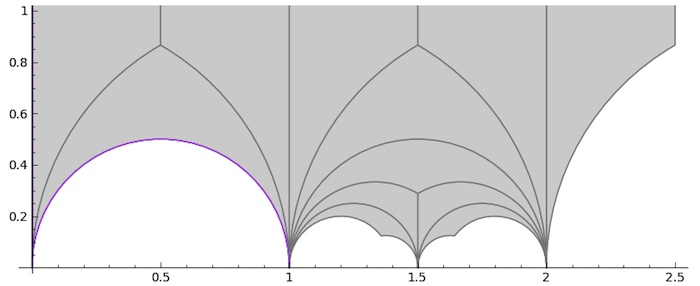# Tag: Sage

A long while ago I promised to take you from the action by the modular group $\Gamma=PSL_2(\mathbb{Z})$ on the lattices at hyperdistance $n$ from the standard orthogonal laatice $L_1$ to the corresponding ‘monstrous’ Grothendieck dessin d’enfant.

Speaking of dessins d’enfant, let me point you to the latest intriguing paper by Yuri I. Manin and Matilde Marcolli, ArXived a few days ago Quantum Statistical Mechanics of the Absolute Galois Group, on how to build a quantum system for the absolute Galois group from dessins d’enfant (more on this, I promise, later).

Where were we?

We’ve seen natural one-to-one correspondences between (a) points on the projective line over $\mathbb{Z}/n\mathbb{Z}$, (b) lattices at hyperdistance $n$ from $L_1$, and (c) coset classes of the congruence subgroup $\Gamma_0(n)$ in $\Gamma$.

How to get from there to a dessin d’enfant?

The short answer is: it’s all in Ravi S. Kulkarni’s paper, “An arithmetic-geometric method in the study of the subgroups of the modular group”, Amer. J. Math 113 (1991) 1053-1135.

It is a complete mystery to me why Tatitscheff, He and McKay don’t mention Kulkarni’s paper in “Cusps, congruence groups and monstrous dessins”. Because all they do (and much more) is in Kulkarni.

I’ve blogged about Kulkarni’s paper years ago:

– In the Dedekind tessalation it was all about assigning special polygons to subgroups of finite index of $\Gamma$.

– In Modular quilts and cuboid tree diagram it did go on assigning (multiple) cuboid trees to a (conjugacy class) of such finite index subgroup.

– In Hyperbolic Mathieu polygons the story continued on a finite-to-one connection between special hyperbolic polygons and cuboid trees.

– In Farey codes it was shown how to encode such polygons by a Farey-sequence.

– In Generators of modular subgroups it was shown how to get generators of the finite index subgroups from this Farey sequence.

The modular group is a free product
$\Gamma = C_2 \ast C_2 = \langle s,u~|~s^2=1=u^3 \rangle$
with lifts of $s$ and $u$ to $SL_2(\mathbb{Z})$ given by the matrices
$S=\begin{bmatrix} 0 & -1 \\ 1 & 0 \end{bmatrix},~\qquad U= \begin{bmatrix} 0 & -1 \\ 1 & -1 \end{bmatrix}$

As a result, any permutation representation of $\Gamma$ on a set $E$ can be represented by a $2$-coloured graph (with black and white vertices) and edges corresponding to the elements of the set $E$.

Each white vertex has two (or one) edges connected to it and every black vertex has three (or one). These edges are the elements of $E$ permuted by $s$ (for white vertices) and $u$ (for black ones), the order of the 3-cycle determined by going counterclockwise round the vertex.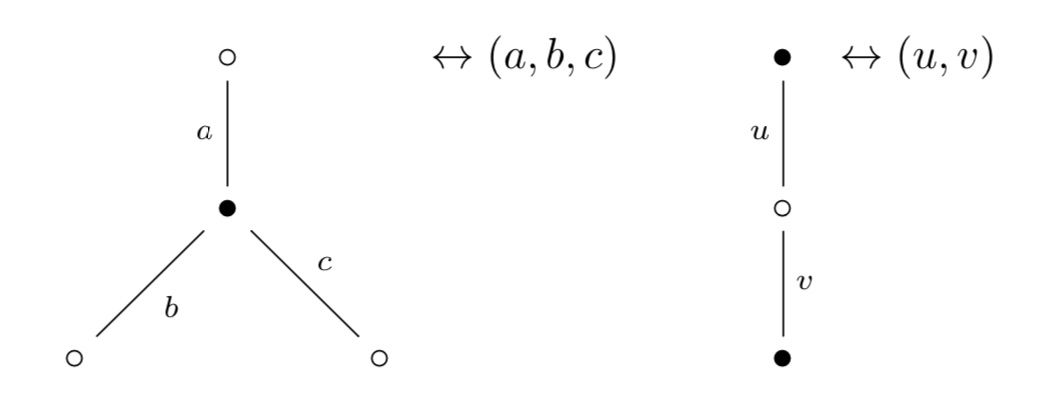Clearly, if there’s just one edge connected to a vertex, it gives a fixed point (or 1-cycle) in the corresponding permutation.

The ‘monstrous dessin’ for the congruence subgroup $\Gamma_0(n)$ is the picture one gets from the permutation $\Gamma$-action on the points of $\mathbb{P}^1(\mathbb{Z}/n \mathbb{Z})$, or equivalently, on the coset classes or on the lattices at hyperdistance $n$.

Kulkarni’s paper (or the blogposts above) tell you how to get at this picture starting from a fundamental domain of $\Gamma_0(n)$ acting on teh upper half-plane by Moebius transformations.

Sage gives a nice image of this fundamental domain via the command

 FareySymbol(Gamma0(n)).fundamental_domain() 

Here’s the image for $n=6$: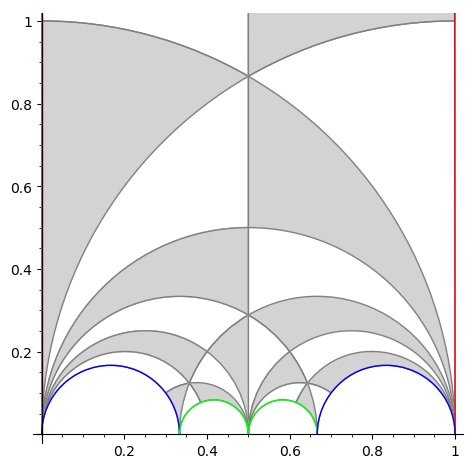The boundary points (on the halflines through $0$ and $1$ and the $4$ half-circles need to be identified which is indicaed by matching colours. So the 2 halflines are identified as are the two blue (and green) half-circles (in opposite direction).

To get the dessin from this, let’s first look at the interior points. A white vertex is a point in the interior where two black and two white tiles meet, a black vertex corresponds to an interior points where three black and three white tiles meet.

Points on the boundary where tiles meet are coloured red, and after identification two of these reds give one white or black vertex. Here’s the intermediate picture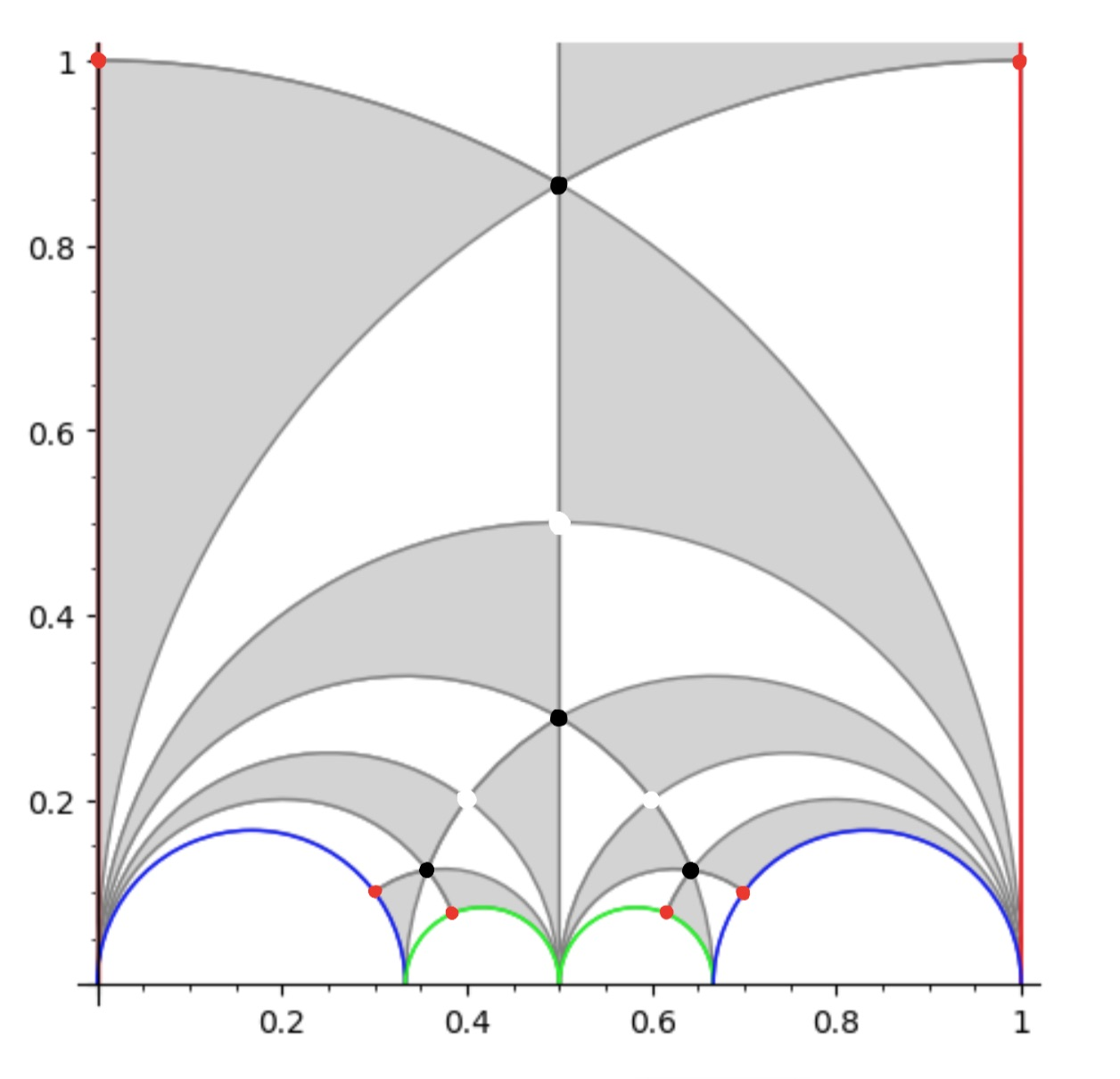The two top red points are identified giving a white vertex as do the two reds on the blue half-circles and the two reds on the green half-circles, because after identification two black and two white tiles meet there.

This then gives us the ‘monstrous’ modular dessin for $n=6$ of the Tatitscheff, He and McKay paper: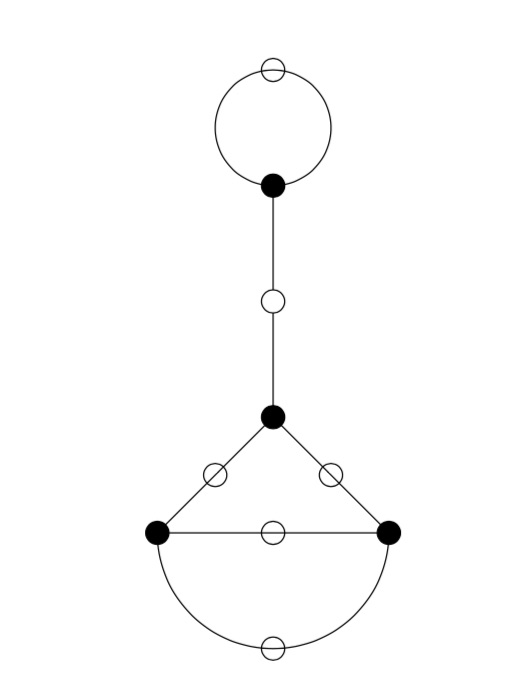Let’s try a more difficult example: $n=12$. Sage gives us as fundamental domain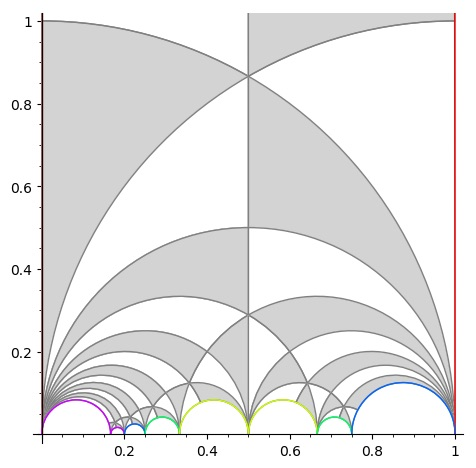giving us the intermediate picture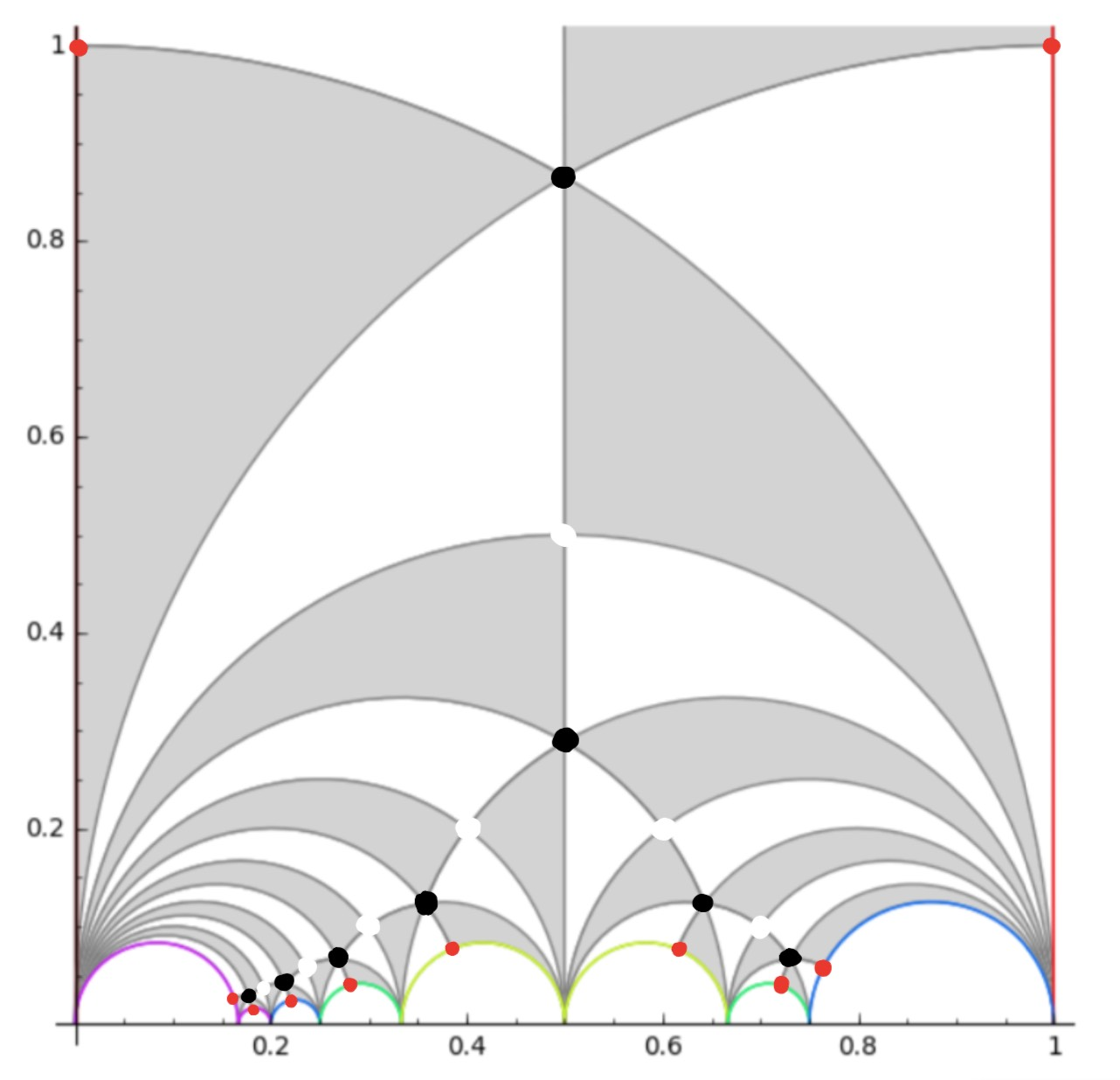and spotting the correct identifications, this gives us the ‘monstrous’ dessin for $\Gamma_0(12)$ from the THM-paper: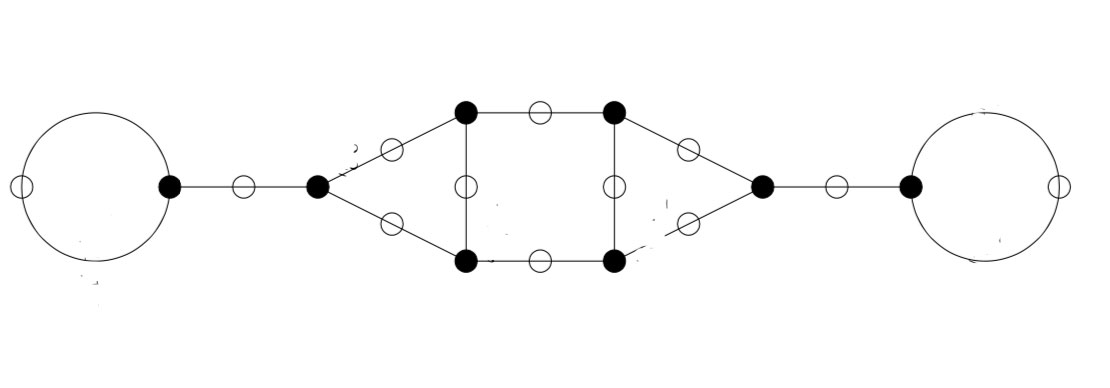In general there are several of these 2-coloured graphs giving the same permutation representation, so the obtained ‘monstrous dessin’ depends on the choice of fundamental domain.

You’ll have noticed that the domain for $\Gamma_0(6)$ was symmetric, whereas the one Sage provides for $\Gamma_0(12)$ is not.

This is caused by Sage using the Farey-code
$\xymatrix{ 0 \ar@{-}[r]_1 & \frac{1}{6} \ar@{-}[r]_1 & \frac{1}{5} \ar@{-}[r]_2 & \frac{1}{4} \ar@{-}[r]_3 & \frac{1}{3} \ar@{-}[r]_4 & \frac{1}{2} \ar@{-}[r]_4 & \frac{2}{3} \ar@{-}[r]_3 & \frac{3}{4} \ar@{-}[r]_2 & 1}$

One of the nice results from Kulkarni’s paper is that for any $n$ there is a symmetric Farey-code, giving a perfectly symmetric fundamental domain for $\Gamma_0(n)$. For $n=12$ this symmetric code is

$\xymatrix{ 0 \ar@{-}[r]_1 & \frac{1}{6} \ar@{-}[r]_2 & \frac{1}{4} \ar@{-}[r]_3 & \frac{1}{3} \ar@{-}[r]_4 & \frac{1}{2} \ar@{-}[r]_4 & \frac{2}{3} \ar@{-}[r]_3 & \frac{3}{4} \ar@{-}[r]_2 & \frac{5}{6} \ar@{-}[r]_1 & 1}$

It would be nice to see whether using these symmetric Farey-codes gives other ‘monstrous dessins’ than in the THM-paper.

Remains to identify the edges in the dessin with the lattices at hyperdistance $n$ from $L_1$.

Using the tricks from the previous post it is quite easy to check that for any $n$ the monstrous dessin for $\Gamma_0(n)$ starts off with the lattices $L_{M,\frac{g}{h}} = M,\frac{g}{h}$ as below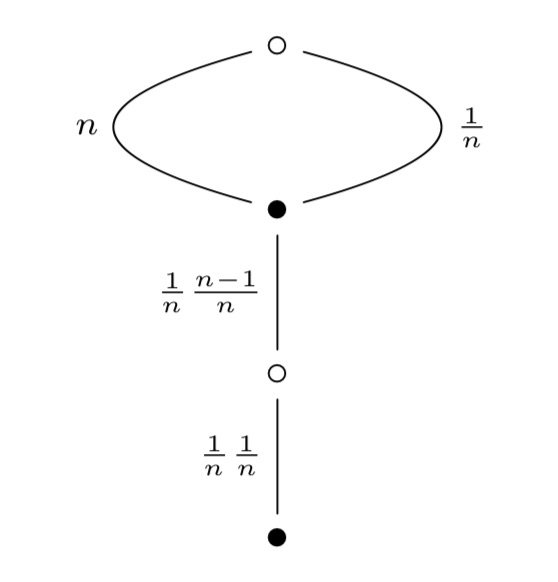Let’s do a sample computation showing that the action of $s$ on $L_n$ gives $L_{\frac{1}{n}}$:

$L_n.s = \begin{bmatrix} n & 0 \\ 0 & 1 \end{bmatrix} \begin{bmatrix} 0 & -1 \\ 1 & 0 \end{bmatrix} = \begin{bmatrix} 0 & -n \\ 1 & 0 \end{bmatrix}$

and then, as last time, to determine the class of the lattice spanned by the rows of this matrix we have to compute

$\begin{bmatrix} 0 & -1 \\ 1 & 0 \end{bmatrix} \begin{bmatrix} 0 & -n \\ 1 & 0 \end{bmatrix} = \begin{bmatrix} -1 & 0 \\ 0 & -n \end{bmatrix}$

which is class $L_{\frac{1}{n}}$. And similarly for the other edges.

Chomp is a 2-player game, usually played with chocolate bars.

The players take turns in choosing one chocolate block and “eat it”, together with all other blocks that are below it and to its right. There is a catch: the top left block contains poison, so the first player forced to eat it dies, that is, looses the game.

If you start with a rectangular bar, the first player has a winning strategy, though it may take you too long to actually find the correct first move. See this post for the strategy-stealing argument.

If you label the blocks of the rectangular bar by $(a,b)$ with $0 \leq a \leq k$ and $0 \leq b \leq l$, with the poisonous one being $(0,0)$, then this can be viewed as choosing a divisor $d$ of $N=p^k q^l$ and removing all multiples of $d$ from the set of divisors of $N$. The first person forced to name $1$ looses.

This allows for higher dimensional versions of Chomp.

If you start with the set of all divisors of a given natural number $N$, then the strategy-stealing argument shows that the first player has a winning move.

A general position of the game corresponds to a finite set of integers, closed under taking divisors. At each move the player has to choose an element of this set and remove it as well as all its multiples.

The thread of $(N|1)$, relevant in understanding a moonshine group of the form $(n|m)+e,f,\dots$ with $N=n \times h$, consists of all divisors of $N$.

But then, the union of all threads for all 171 moonshine groups is a position in higher dimensional Chomp.

Who wins starting from this moonshine thread?

Perhaps not terribly important, but it forces one to imagine the subgraph of the monstrous moonshine picture on the $97$ number-lattices way better than by its Hasse diagram.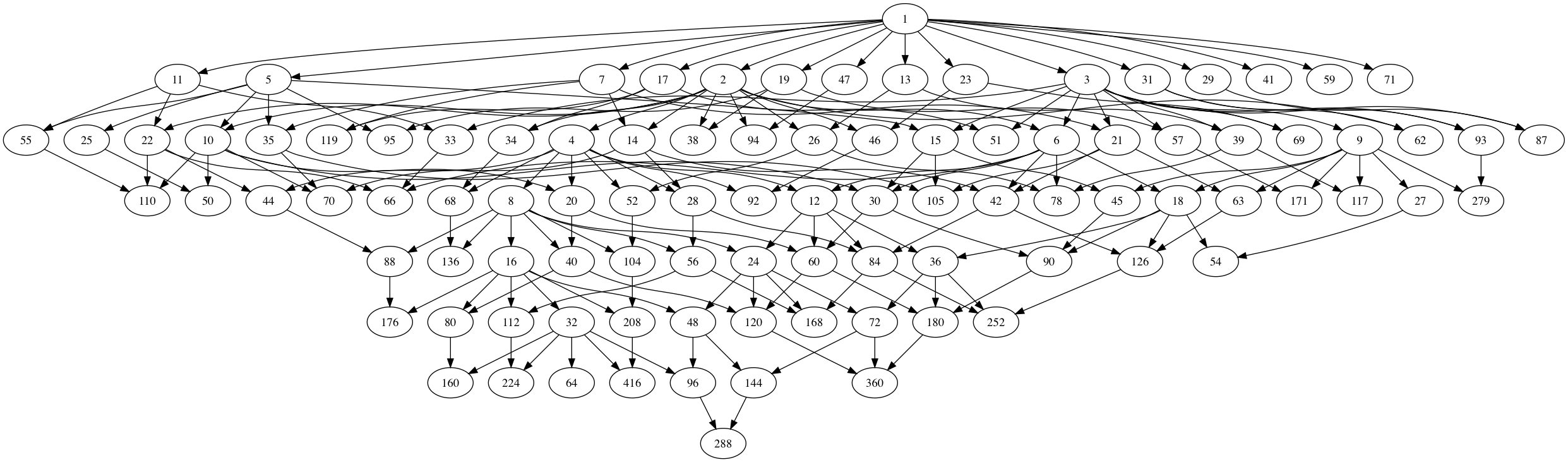Click on the image for a larger version.

By the way, notice the (slight) resemblance with the ‘monstrous moonshine painting’ by AtriaHere’s how the Hasse diagram of the moonshine thread was produced. These are ‘notes to self’, because I tend to forget such things quickly.

1. Work though the list of 171 moonshine groups in Monstrous Moonshine, pages 327-329. Add to a list all divisors of $N$ for a group of type $N+e,f,\dots$ or $n|h+e,f,\dots$ with $N=n \times h$. This should give you these $97$ integers:

1,2,3,4,5,6,7,8,9,10,11,12,13,14,15,16,17,18,19,20,21,22,23,24,25,26,27,28,29,30,
31,32,33,34,35,36,38,39,40,41,42,44,45,46,47,48,50,51,52,54,55,56,57,59,60,62,
63,64,66,68,69,70,71,72,78,80,84,87,88,90,92,93,94,95,96,104,105,110,112,117,
119,120,126,136,144,160,168,171,176,180,208,224,252,279,288,360,416

2. Let $L$ be this list and use Sage:

P=Poset((L,attrcall("divides")),linear_extension=True) H=P.hasse_diagram() H.graphviz_string() 

3. Copy the output to a file, say chomp.dot, and remove all new-line breaks from it.

4. Install Graphviz on Mac OS X.

5. In Terminal, type
dot -Tpng chomp.dot -o chomp.png

The sporadic second Janko group $J_2$ is generated by an element of order two and one of order three and hence is a quotient of the modular group $PSL_2(\mathbb{Z}) = C_2 \ast C_3$.

This Janko group has a 100-dimensional permutation representation and hence there is an index 100 subgroup $G$ of the modular group such that the fundamental domain $\mathbb{H}/G$ for the action of $G$ on the upper-half plane by Moebius transformations consists of 100 triangles in the Dedekind tessellation.

Four years ago i tried to depict this fundamental domain in the Farey symbols of sporadic groups-post using Chris Kurth’s kfarey package in Sage, but the result was rather disappointing.

Now, the kfarey-package has been greatly extended by Hartmut Monien of Bonn University and is integrated in the latest version of Sage, SAGE 5.0, released a few weeks ago.

Using the Farey symbol sage-documentation it is easy to repeat the calculations from four years ago and, this time, we do obtain this rather nice picture of the fundamental domain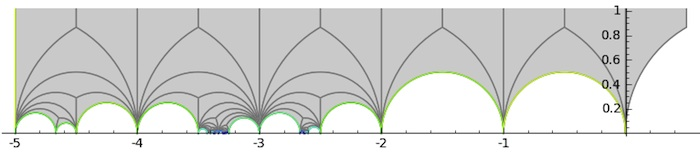But, there’s a lot more one can do with the new package. By combining the .fractions() with the .pairings() info it is now possible to get the corresponding Farey code which consists of 34 edges, starting off with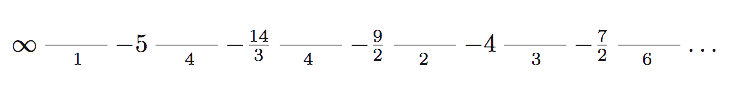Perhaps surprisingly (?) $G$ turns out to be a genus zero modular subgroup. Naturally, i couldn’t resist drawing the fundamental domain for the 12-dimensional permutations representation of the Mathieu group $M_{12}$ and compare it with that of last time.